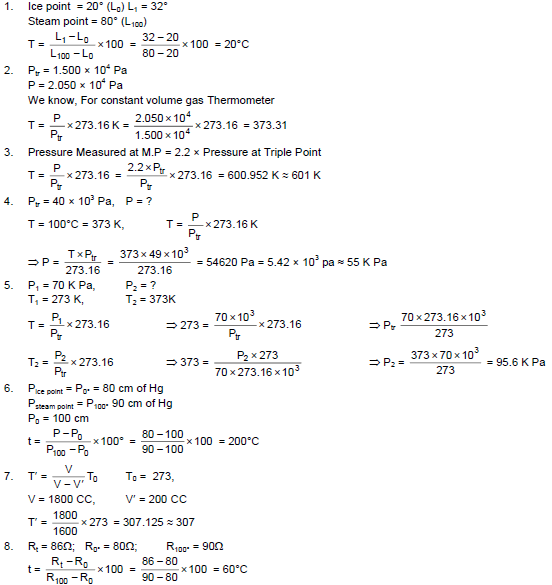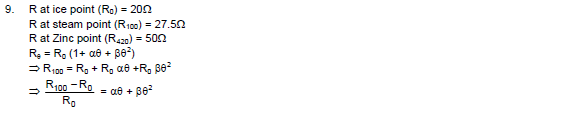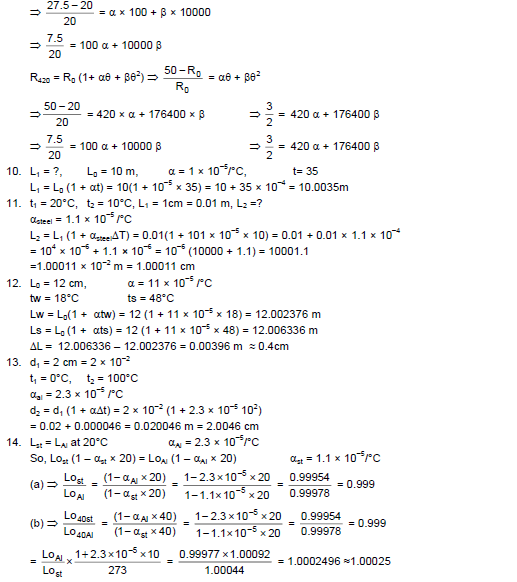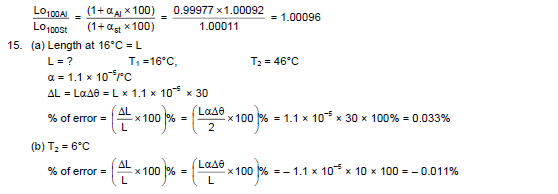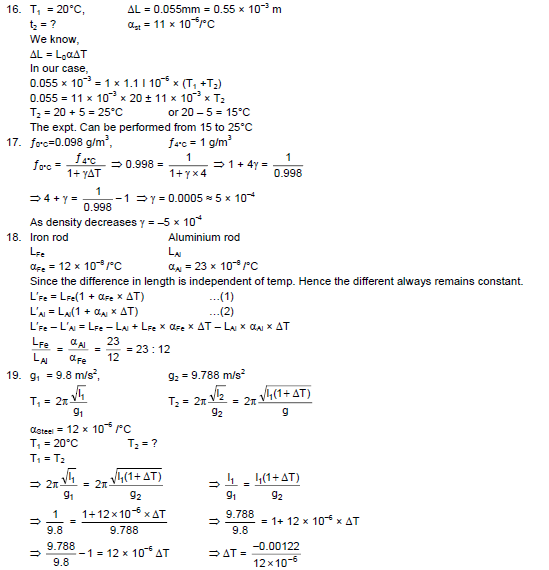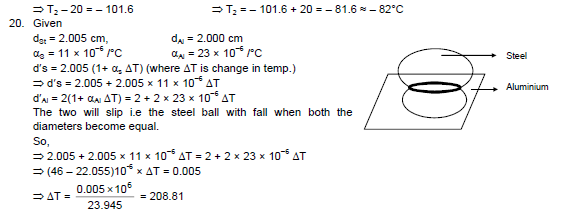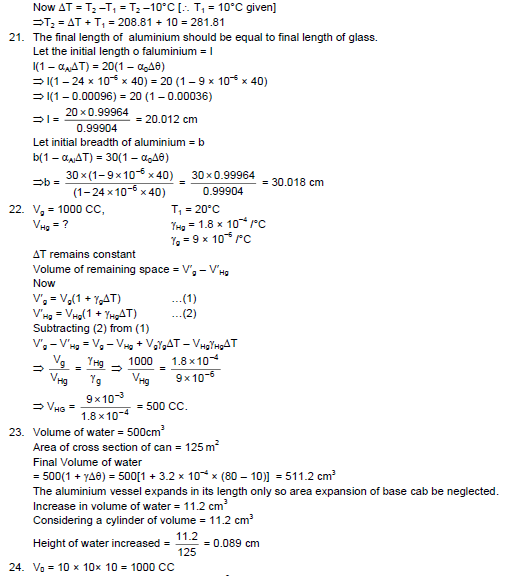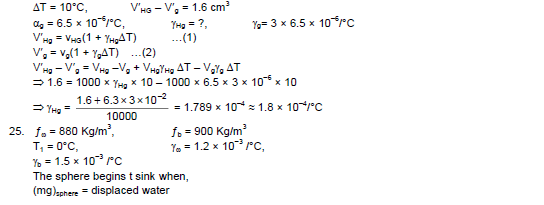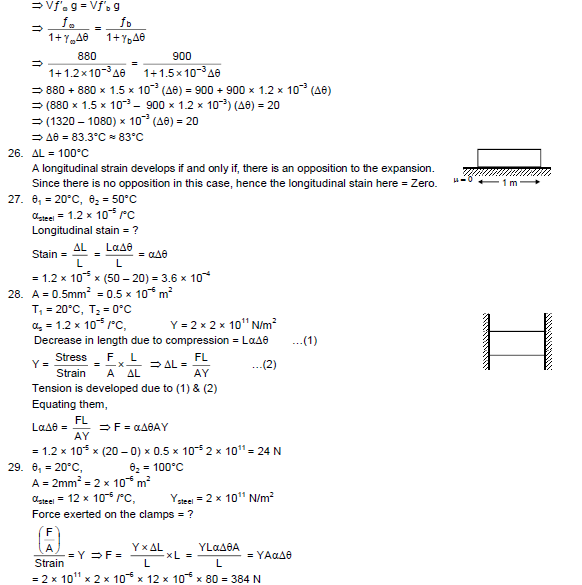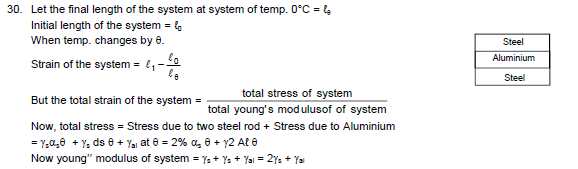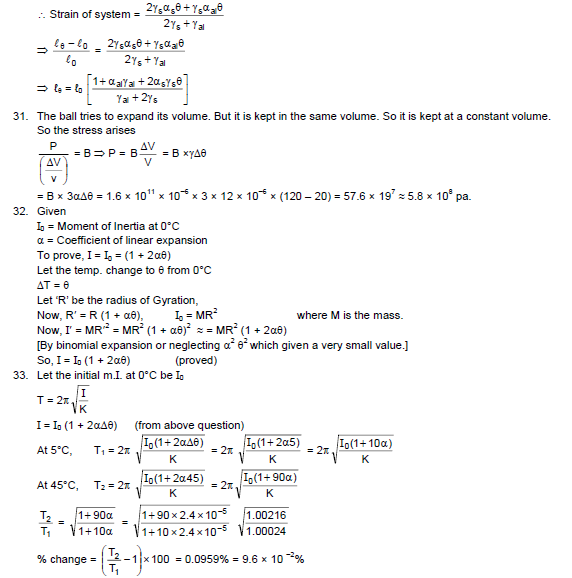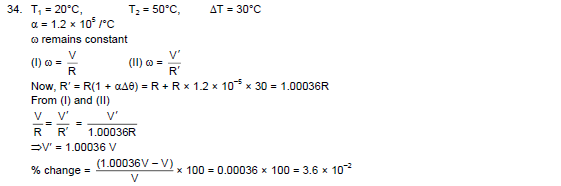# HC Verma Solutions Vol 2 Chapter 1 Heat and Temperature

HC Verma Solutions Vol 2 Chapter 1 Heat and Temperature is provided here to help students understand the topics more clearly. Usually, before working on any exercise it is important to understand the key concepts and terminologies. Therefore the solutions are designed to help students learn more about the chapter topics and also tackle difficult questions. The chapter deals with the topic of heat which can be defined as the degree or level of hotness/ coldness of an object or environment. It is the transfer of energy from a hotter system to a cooler system due to a temperature difference between them. The SI unit of heat is joule (J). A systems temperature rises when the heat is transferred to it. Temperature is measured in degree Celsius, Kelvin or Fahrenheit.

In addition to understanding the concepts, the chapter also contains important exercises that students should solve and practice. These exercises are also extremely important as it gives students an idea to tackle complex problems based on the concepts related to Heat and Temperature. The HC Verma solutions further contain answers to all the exercises which students can refer and study effectively for their final exam and various competitive exams like JEE, NEET, etc.

## Heat And Temperature Main Topics

Some of the exercises are based on topics such as;

• The relation between Heat and Temperature
• Thermal equilibrium
• The relation between Celsius and Fahrenheit, Kelvin
• Ice point
• Steam point
• Triple point
• Constant Volume gas thermometer
• Change of moment of inertia with temperature
• The coefficient of linear expansion

Physics is a branch of science and is the basis of future technology. Students who aspire to excel in the developing field of science and technology should have strong basics in physics. These Solutions will help students master the concepts and enhance their problem-solving skills. Get solutions of HC Verma book for Class 12 below.

## Important Questions In Chapter 1

1. Can two bodies be in thermal equilibrium if they are not in contact? Explain

2. When a glass bottle with a tightly closed metal lid is put in hot water for some time, it can be opened more easily. Why does this happen?

3. We take a metal sheet and make a circular hole in it. Then the sheet is heated. What will happen to the hole?

(a) The hole increases and becomes bigger (b) Becomes smaller (c) no changes in size (d) Is deformed.

4. Suppose the temperature at the surface of a lake is 4°C. The temperature of water at the bottom can be;

(a) 0°C (b) 4°C (c) 6°C (d) 9°C.

5. Mark the correct pair or pairs that may give equal numerical values for the temperature of a body?

(a) Platinum and Kelvin (b) Celsius and Kelvin (c) Kelvin and Fahrenheit

## HC Verma Solutions Vol 2 Heat and Temperature Chapter 1